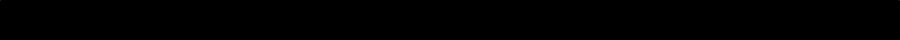Aerofluids.org
Humberto Medina$$p=\rho RT$$
$$\tau_w = \mu \frac{\partial u}{\partial y}$$